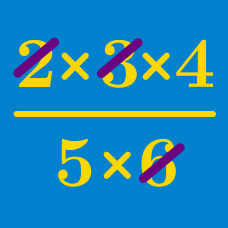Algebra

# Order of Operations

Evaluate $5 + 4 - 2 + 3 - 1 + 6.$

True or False?

$3 + 3 + 3 \div 3 = 3$

Evaluate $2 \times 3 \div 6 \times 5 \times 4 \div 10.$

Which of the following is correct?

$$~~(a)~~ 4 + 2 \times 3 = 18$$
$$~~(b)~~ 10 \div 2 - 3 = 2$$
$$~~(c)~~ 4 \times 4 \div 4 \times 4 = 1$$
$$~~(d)~~ 15 - 3 \div 3 + 10 = 14$$

Evaluate $9 - 3 \times 2.$

×